Study P3 Mathematics Multiplication and Division - Geniebook# Multiplication And Division

Addition, subtraction, division and multiplication are the primary arithmetic operations which allow us to add, subtract, divide or multiply two or more numbers or any mathematical values. The symbol which represents multiplication is ‘$$\times$$’ and division is ‘$$\div$$‘. These arithmetic operations are performed on real numbers.

## Multiplication And Division

1. Multiplication Tables of  6, 7, 8 and 9
2. Understanding multiplication

## 1. Multiplication Tables 6, 7, 8 And 9For the multiplication table of 6, we count on by 6.

There is an increase of 6 from one number to the next.

For the multiplication table of 7, we count on by 7.

There is an increase of 7 from one number to the next.For the multiplication table of 8, we count on by 8.

There is an increase of 8 from one number to the next.

For the multiplication table of 9, we count on by 9.

There is an increase of 9 from one number to the next.

#### A way to remember the multiplication table of 9

• Step 1: Write 0 to 9 in ascending order on the left side.
 Left 0 1 2 3 4 5 6 7 8 9

• Step 2: Write 9 to 0 in descending order on the right side.
 Left Right 0 9 1 8 2 7 3 6 4 5 5 4 6 3 7 2 8 1 9 0

Question 1:

Match.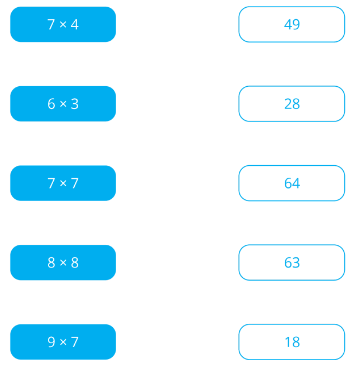Solution: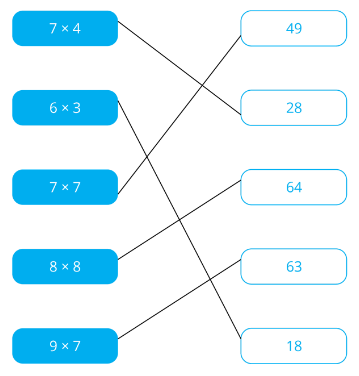Question 2:

Do the following.

$$6 \times 7 = \text{__________}$$

Which of the following is the correct answer?

1. 42
2. 49
3. 56
4. 67

Solution:

$$6 \times 7 = 42$$

(1) 42

Question 3:

Do the following.

$$9 \times 3 = \text{__________}$$

Solution:

$$9 \times 3 = 27$$

27

Question 4:

Do the following.

$$6 \times 6 = \text{__________}$$

Solution:

$$6 \times 6 = 36$$

36

Question 5:

Do the following.

$$8 \times 6 = \text{__________}$$

Solution:

$$8 \times 6 = 48$$

48

Question 6:

Do the following.

$$9 \times 9 = \text{__________}$$

Solution:

$$9 \times 9 = 81$$

81

Question 7:

Which of the following rockets shows the correct expression to the answer on the moon?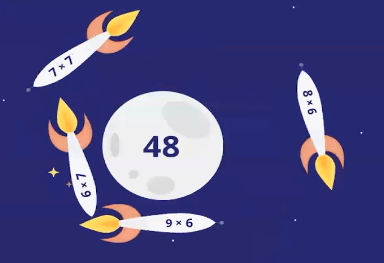Solution:

$$8 \times 6 = 48$$

$$8 \times 6$$

## 2. Understanding Multiplication

Recall that multiplication is the adding of equal groups.

Let’s take a look at the following:

Example: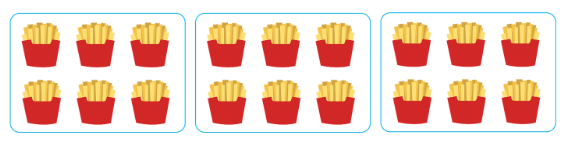Solution:

There are 3 groups of fries.

In each group, there are 6 packets of fries.

\begin{align} 6 + 6 + 6 &= 18\\[2ex] 3 \text{ groups of } 6 &= 18 \\[2ex] 3 \times 6 &= 18 \end{align}

There are 18 packets of fries altogether.

18 packets

Question 1:

Rearrange the numbers and symbols to form an equation that best represents the following: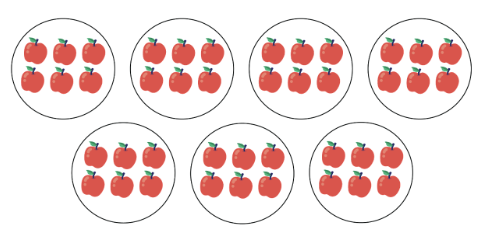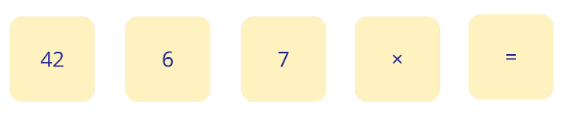Solution:

There are 7 groups of apples.

In each group, there are 6 pieces of apples.

\begin{align} 7 \text{ groups of } 6 \text{ apples} &= 42 \\[2ex] 7 \times 6 &= 42 \end{align}

$$7 \times 6 = 42$$

Question 2:

Which of the following best represents the picture?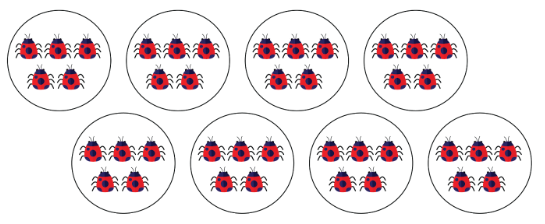1. $$5 \times 5 = 25$$
2. $$4 \times 10 = 40$$
3. $$8 \times 5 = 40$$
4. $$8 \times 8 = 64$$

Solution:

There are 8 groups of beetles.

In each group, there are 5 beetles.

\begin{align} 8\text{ groups of } 5 \text{ beetles} &= 40 \\[2ex] 8 \times 5 &= 40 \end{align}

(3) $$8 \times 5 = 40$$

Question 3:

Which of the following best represents the picture?1. $$4 \times 4 = 16$$
2. $$9 \times 4 = 36$$
3. $$6 \times 6 = 36$$
4. $$4 \times 10 = 40$$

Solution:

There are 9 groups of balls.

In each group, there are 4 balls.

\begin{align} 9 \text{ groups of } 4 \text{ balls} &= 36 \\[2ex] 9 \times 4 &= 36 \end{align}

(2) $$9 \times 4 = 36$$

Question 4:

What is the least number of stars that must be added to the figure below so that all the stars can be put into groups of 8 exactly?1. 5
2. 6
3. 8
4. 4

Solution:

Total number of stars at first $$= 18$$

Multiplication table of 8: 8, 16, 24, …

Total number of stars in the end $$= 24$$
Number of stars that must be added\begin{align}\\[2ex] &=24-18\\[2ex] &=6 \end{align}

(2) 6

Question 5:

Fill in the blank:

$$4 + 4 + 4 + 4 + 4 + 4 + 4 = \text{__________} \times 4$$

Solution:

\underbrace{4 + 4 + 4 + 4 + 4 + 4 + 4 }_{\begin{align}\\ 7 \text{ groups of } 4 \; &= \;7 \;\times\; 4 \end{align}} = \text{__________} \times 4

$$4 + 4 + 4 + 4 + 4 + 4 + 4 = 7 \times 4$$

7

Question 6:

Fill in the blank.

$$7 + 7 + 7 + 7 + 7 + 7 = \text{__________} \times 7$$

Solution:

\underbrace{7 + 7 + 7 + 7 + 7 + 7 }_{\begin{align}\\ 6 \text{ groups of } 7 \; &= \;6 \;\times\; 7 \end{align}} = \text{__________} \times 7

6

Question 7:

$$6 \times 9$$ is the same as $$\text{__________}$$.

1. $$9 + 9 + 9 + 9 + 9 + 9$$
2. $$9 + 9 + 9$$
3. $$9 \times 9 \times 9 \times 9 \times 9 \times 9$$
4. $$6 \times 6 \times 6 \times 6 \times 6 \times 6 \times 6 \times 6 \times 6$$

Solution:

\begin{align} 6 \times 9 &= 6 \text{ groups of } 9\\[2ex] &= 9 + 9 + 9 + 9 + 9 + 9 \end{align}

(1) $$9 + 9 + 9 + 9 + 9 + 9$$

Question 8:

Fill in the blank.

$$5 \times 8 = 4 \text{ groups of } 8 + \text{__________ group(s) of } 8$$

Solution:

\begin{align} 5 \times 8 &= 5 \text{ groups of } 8\\[2ex] 5 \text{ groups of } 8 &= 4 \text{ groups of } 8 + \text{__________ group(s) of } 8\\[4ex] 5 - 4 &= 1\\[2ex] 5 \text{ groups of } 8 &= 4 \text{ groups of } 8 + 1 \text{ group(s) of } 8 \end{align}

1

Question 9:

Fill in the blank.

$$8 \times 6 = 6 \text{ groups of } 6 + \text{__________ groups of } 6$$

Solution:

\begin{align} 8 \times 6 &= 8 \text{ groups of } 6\\[2ex] 8 \text{ groups of } 6 &= 6 \text{ groups of } 6 + \text{ __________ groups of } 6\\[4ex] 8 - 6 &= 2\\[2ex] 8 \text{ groups of } 6 &= 6 \text{ groups of } 6 + 2 \text{ groups of } 6 \end{align}

2

Question 10:

Fill in the blank.

$$9 \times 7 = 5 \times 7 + \text{__________} \times 7$$

Solution:

\begin{align} 9 \times 7 &= 9 \text{ groups of } 7\\[2ex] 9 \text{ groups of } 7 &= 5 \text{ groups of } 7 + \text{ __________ groups of } 7\\[4ex] 9 - 5 &= 4\\[2ex] 9 \text{ groups of } 7 &= 5 \text{ groups of } 7 + 4 \text{ groups of } 7 \end{align}

4

Question 11:

Fill in the blank.

$$4 \times 7 = 9 \text{ groups of } 7 - \text{ __________ groups of } 7$$

Solution:

\begin{align} 4 \times 7 &= 4 \text{ groups of } 7\\[2ex] 4 \text{ groups of } 7 &= 9 \text{ groups of } 7 - \text{ __________ groups of } 7\\[4ex] 9 - 4 &= 5\\[2ex] 4 \text{ groups of } 7 &= 9 \text{ groups of } 7 - 5 \text{ groups of } 7 \end{align}

5

Question 12:

Fill in the blank.

$$8 \times 9 = 10 \text{ groups of } 9 - \text{__________ groups of } 9$$

Solution:

\begin{align} 8 \times 9 &= 8 \text{ groups of } 9\\[2ex] 8 \text{ groups of } 9 &= 10 \text{ groups of } 9 - \text{ __________ groups of } 9\\[4ex] 10 - 8 &= 2\\[2ex] 8 \text{ groups of } 9 &= 10 \text{ groups of } 9 - 2 \text{ groups of } 9 \end{align}

2

Question 13:

Fill in the blank.

$$9 \times 6 = 10 \times 6 - \text{__________} \times 6$$

Solution:

\begin{align} 9 \times 6 &= 9 \text{ groups of } 6\\[2ex] 9 \text{ groups of } 6 &= 10 \text{ groups of } 6 - \text{__________ group of } 6\\[4ex] 10 - 9 &= 1\\[2ex] 9 \text{ groups of } 6 &= 10 \text{ groups of } 6 - 1 \text{ group of } 6 \end{align}

1

Question 14:

I am a number between 40 and 60. You can find me in the multiplication tables of 6 and 9. What number am I?

1. 36
2. 48
3. 54
4. 56

Solution:

Let’s take a look at the multiplication tables of 6 and 9.

Multiplication tables of 6: 6, 12, 18, 24, 30, 36, $$42, 48, 54$$, 60

Multiplication tables of 9: 9, 18, 27, 36, $$45, 54$$, 63, 72, 81, 90

The highlighted numbers are the numbers between 40 and 60.

The number 54 is in both the multiplication tables of 6 and 9.

(3) 54

Question 15:

I am a number between 20 and 40. You can find me in the multiplication tables of 3, 6 and 8. What number am I?

Solution:

Let’s take a look at the multiplication tables of 3, 6 and 8.

Multiplication tables of 3: 3, 6, 9, 12, 15, 18, $$21, 24, 27, 30$$

Multiplication tables of 6: 6, 12, 18, $$24, 30, 36,$$ 42, 48, 54, 60

Multiplication tables of 8: 8, 16, $$24, 32, 40$$, 48, 56, 64, 72, 80

The highlighted numbers are the numbers between 20 and 40.

The number 24 is in the multiplication tables of 3, 6 and 8.

24

Continue Learning
Volume Money
Mass Fractions
Addition And Subtraction Whole Numbers 1
Whole Numbers 3 Whole Numbers 6
Bar Graphs Angles
Multiplication And Division Length, Mass And Volume
Length Area And Perimeter
Parallel And Perpendicular LinesPrimaryPrimary 1Primary 2Primary 3EnglishMaths
Volume
Money
Mass
Fractions
Whole Numbers 1
Whole Numbers 3
Whole Numbers 6
Bar Graphs
Angles
Multiplication and Division
Length, Mass And Volume
Length
Area and Perimeter
Parallel And Perpendicular LinesSciencePrimary 4Primary 5Primary 6SecondarySecondary 1Secondary 2Secondary 3Secondary 4